# IB Manual – Estimating Free Cash Flow

How to forecast money coming into the company

## Estimating Free Cash Flow

To arrive at a Discounted Cash Flow (DCF) valuation, we split our model into a visible forecast period (typically 3 to 5 years) and terminal value period.  Both of these segments of the model require estimating free cash flow in the future.

### Why discounted cash flows?

We are interested in estimating free cash flow as the money coming into the firm, available for use, because it is the best measure of a firm’s value. Earnings can be manipulated in terms of revenue and cost recognition and much of the work of adjustments with comps valuation is spent trying to “clean” the earnings information. In the end, “cash is fact – profit is an opinion” (The Economist, August 2, 1997).### Estimating free cash flow – Which cash flows do we use?

For valuation purposes, we do not want to use operating cash flow. The following provides an example of operating cash flow derivation for valuation: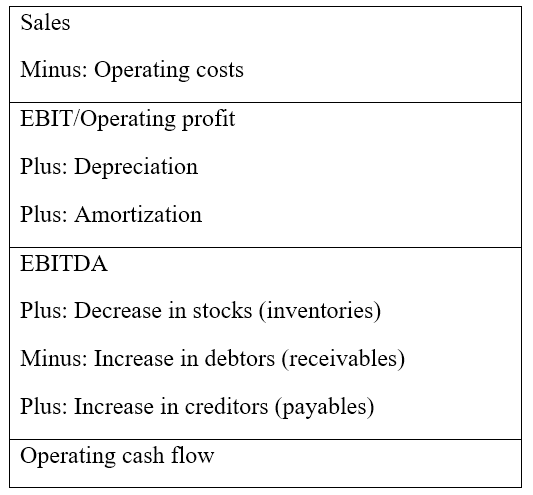The derivation above considers the investment required to maintain existing cash flows and support future cash flows.

### FCF to firm vs FCF to equity

Free Cash Flow to the Firm (FCFF) is:

“The amount of cash that a company has left over after it has paid all of its expenses, but before any payments or receipts of interest or dividends, before any payments to or from providers of capital, and adjusting tax paid to what it would have been if the company had no cash or debt.”

Note that an FCF valuation valuing the whole company (firm value) differs from one that produces an equity valuation. Free cash flow to equity (FCFE) creates an equity valuation and is the cash claim left to equity shareholders after all expenses are paid. In contrast, free cash flow to the firm (FCFF) creates a company valuation and is the potential cash claims left to all providers of finance after all expenses are paid. The main difference between FCFE and FCFF is the interest paid (net of tax) to debt finance providers. FCFF is before interest.

The following is a breakdown of the calculation for FCFF and FCFE: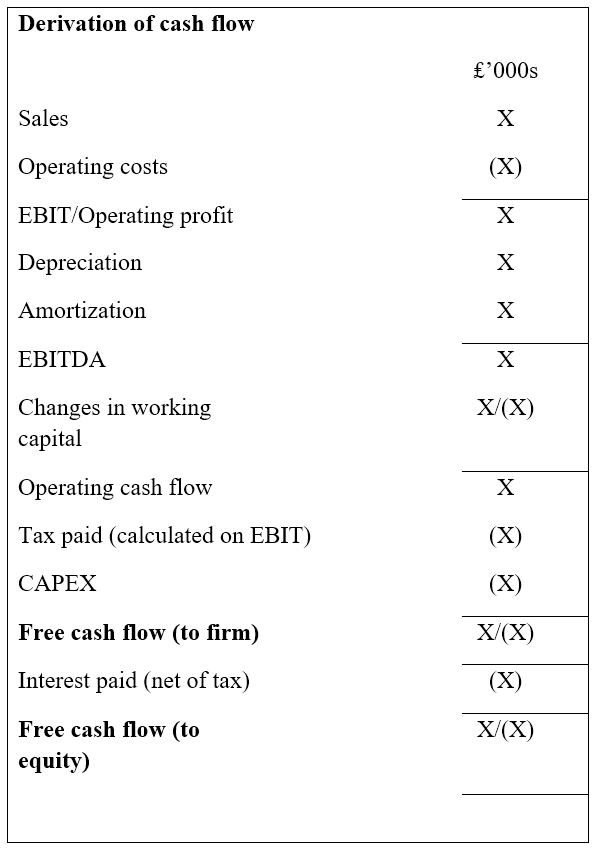### The key value drivers of free cash flow

To create a forecast cash flow, we need to understand how a business operates. How does a business create value? To properly model, we need to understand what drives cash growth.

Key drivers of Free Cash Flows are:

1. Sales growth rates
2. EBITDA margins
3. Cash tax rates
4. Fixed capital investment or capital expenditure
5. Working capital requirements

The analysis examines each of these key drivers.

#### #1 Sales growth rates

Without sales, there is no business or cash. To analyze sales growth rates, one must analyze current sales activity, recent investment activity, and working capital levels to determine if future growth is possible. Then, growth analysis rates need to be adjusted for:

• Market information
• Strategic considerations
• Pricing policy decisions
• The economy
• Competition (and barriers to entry)

#### #2 EBIT and EBITDA margins

Margins reflect how much of its earnings a company can retain while conducting business. Note that margins will depend on the industry, as well as on what sales are made (high-profit goods vs low-profit goods). A machinery company with high fixed costs will report different margins and growth rates from a small technology start-up. Margins allow valuation models to go from sales forecasts to profit figures.

#### #3 Cash tax rate

Cash tax rate is a key driver, as it affects previously incurred tax losses and deferred taxation. In modeling, cash tax rates are driven through EBIT or EBITA (treating the depreciation charge as an approximation to the allowed tax depreciation deduction), with effective cash tax rates adjusted for all other tax adjustments.

#### #4/5 Fixed and working capital requirements

Again, the main difference between operating cash flow and cash flows for valuation purposes is the investment necessary to maintain existing cash flows and support future cash flows. Working capital requirements support operations and future growth. For most businesses, working capital investments grow with sales. Fixed capital requirements can be split into two elements:

• Maintenance capital expenditure – The replacement of the existing capital base
• Investment capital expenditure – The investment in new assets to meet forecast growth projections

The source of long-term growth is an investment in capital expenditure. Therefore, higher capital expenditure should be reflected in higher sales growth in the short to medium term.  As the company expands, working capital requirements should expand in response.

### Calculating the terminal value

With the terminal value, we are estimating the continuing period of our cash flow. The terminal value is used so as to not exaggerate forecasted cash flows. This is because business growth typically becomes constant after a given period. Often the terminal value is used to calculate cash flows that extend beyond 5 to 10 years.

To calculate terminal value, one must:

• Select a terminal value calculation method
• Estimate the relevant parameters for the terminal value calculation
• Calculate the terminal value
• Discount the terminal value to present value

The most widely used calculations for terminal value are the DCF method (cash flow perpetuity) and company comparables.

### Estimating free cash flow – DCF method (cash flow perpetuity method)

The DCF method for an FCFF terminal valuation works on the assumption that by the end of the visible cash flow period, FCFF will reach maturity and behave as a perpetuity, like stock valuation. These perpetuities can assume no growth. A DCF terminal value cash flow perpetuity calculation may assume zero cash flow growth.  For an FCFF valuation, it involves dividing the terminal year FCFF by WACC: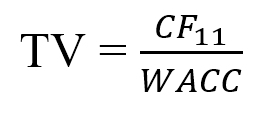Where:

• CF11 – The cash flow in period 11 (where year 10 is the last year of the visible cash flow period)
• WACC – The weighted average cost of capital

The above method is for a simple DCF valuation. However, some firms may argue that growth will continue past the forecast period. For these companies, we use the following: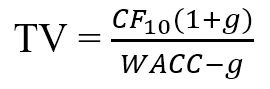Where:

• CF10 – The cash flow in period 10 (where year 10 is the last year of the visible cash flow period)
• G – The perpetuity growth rate (expressed as a % age)
• WACC – The weighted average cost of capital

Keep in mind that this formula runs at an assumed constant growth rate. Furthermore, forecasts for growth 5,10, or 20 years into the future are highly prone to error.

### Key considerations when using the DCF method (Cash flow perpetuity method)

During DCF analysis, consider that:

• The DCF method relies on the cash flow estimated in the final year of the forecast period and must, therefore, be confident in the forecasted numbers and assumptions
• The reliance on the terminal value calculation as the main contributor to the overall valuation can be managed by extending the forecast period
• Growth is easy to exaggerate – Companies will not grow at 8% in perpetuity when GDP growth is 2%

### Value driver methodology

The problem with the above method is that it does not allow for growth in capex or changes in working capital. For most valuations with a steady state GDP growth rate, this is not critical, but as higher growth rates are incorporated into the model, the valuation is likely to be exaggerated.

The value driver formula below addresses the issue: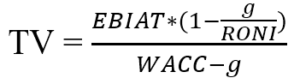Where:

• TV – Terminal value
• EBIAT – Earnings before interest after tax
• g – The growth rate
• RONI – Return on new investment
• WACC – The weighted average cost of capital

### Comps method of computing the terminal value

We are also able to use profit metrics to forecast terminal value for DCF valuation. In an FCFF DCF valuation, the best multiples to use are Firm Value (FV) multiples, as these multiples will give a firm-wide terminal value.

### Using an appropriate multiple

For valuation, the ideal comps to use are the ones for companies with similar business growth and risks. It is believed that the market, on average, prices companies properly. Multiples are often used as a comparison to the terminal value achieved in perpetuity. However, there are disagreements over the validity of comps in valuation. A key issue with comps is that the implied growth rate is not a transparent element of the terminal value calculation, so it is easy to use an inappropriate multiple that implies excessive growth.

### Backing out the implied growth rate of the multiple

“Backing out” the implied growth rate of a multiple can help fix the issue with excessive growth: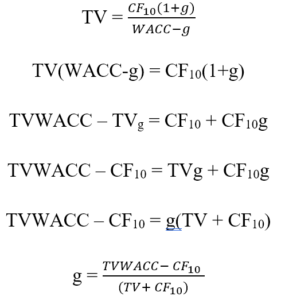Where:

• TV – Terminal value calculated using a multiple
• CF10 – The cash flow in period 10 (where year 10 is the last year of the visible cash flow period)
• g – The perpetuity growth rate (implied)
• WACC – The weighted average cost of capital

Using this methodology, we may determine the implied growth rate based on a multiple.